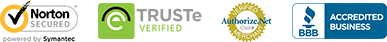## Penguin Balance Math Toy Set

Tactile Activity Set Learn the fundamentals of weight measurement and progress through multiple levels of math learning with this irresistible set of math toys.  The chunky 3-D numerals are weighted proportionally, so 1 & 2 together will balance with 3, and so on.  The mini penguin figures equal the numeral... Read More

Discovery Toys

Out of stockTactile Activity Set

Learn the fundamentals of weight measurement and progress through multiple levels of math learning with this irresistible set of math toys.  The chunky 3-D numerals are weighted proportionally, so 1 & 2 together will balance with 3, and so on.  The mini penguin figures equal the numeral 1 in weight.  Includes deck of 54 cards with a variety of illustrated balancing activities, including: numeral association / counting / numeral identification / addition.  Penguin balance, 2 balance trays, 10 weighted numerals, 19 mini weighted penguins, 54 activity cards, activity guide.

13” x 6.25” x 3.75” / 33 x 16 x 9.5cm.

from 3 years+

Benefits

• Visualize early math concepts with hands-on 3-dimensional objects
• The hands-on penguins and numerals allow the child to easily add to or take away from sets, or to rearrange
• Combining kinesthetic and visual learning will help to reinforce the connections formed in the child's brain, increasing the odds of retaining the learning
• Learn the concept of balance and the relative values of weights with this math toy set
• Associating a numeral with weight reinforces the notion of sequential progression and allows for exploration of math operations
• Practice predicting and experimenting.
• Develops fine motor dexterity.
• Promotes early math learning:
• Weight
• Counting